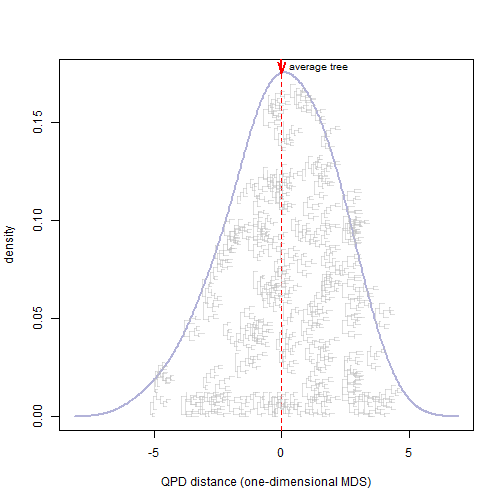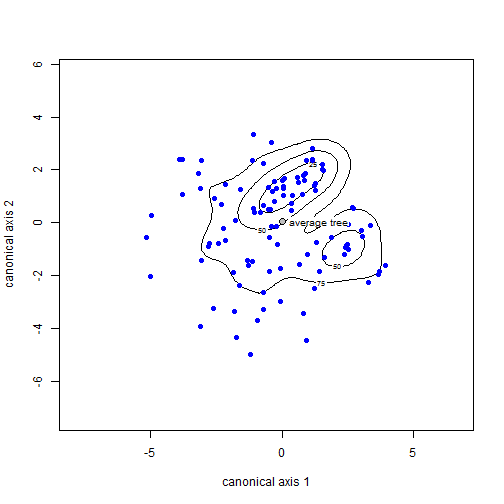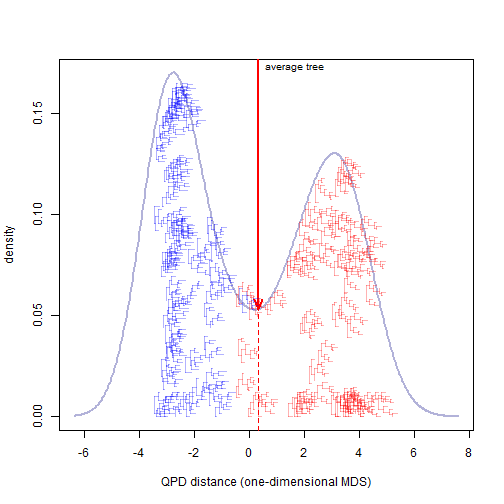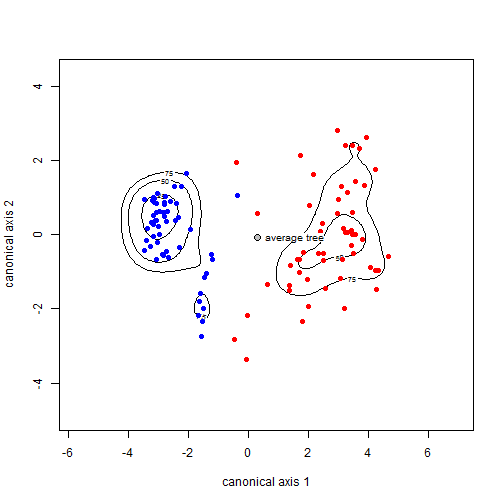## Tuesday, May 10, 2016

### Average trees in 'tree space'

I have just been messing around with visualizing treespace (or, more accurately, a projection of the distances between trees into Euclidean space).

What I'm going to show is a distribution of trees, and the average from this distribution by some measure, projected into a one or two dimensional tree-space. Here I'll use 'quadratic path difference,' which is a measure of the degree to which the implied distances between taxa for two trees differ one from the other. I'm also going to overlay the “average” tree by the same criterion, which can be obtained by computing the least-squares tree from the mean patristic distances implied by the set of input trees.

Here is the result of a couple of experiments.

Firstly, with one set of trees sampled by performing random NNIs from a single starting tree:

``````library(phytools)
library(phangorn)
library(ks)

## helper function to use later
mt<-phytools:::make.transparent

## generate random NNIs
trees<-rNNI(rtree(n=8),moves=sample(1:8,replace=TRUE,size=120))

## compute average tree (to be used later)
ave.tree<-midpoint(ls.consensus(trees))
``````
``````## Best Q = 0.0573618227165727
``````
``````## Solution found after 1 set of nearest neighbor interchanges.
``````
``````## append to the set of simulated trees
ave.tree<-list(ave.tree)
class(ave.tree)<-"multiPhylo"
trees<-c(trees,ave.tree)

## compute all pairwise "quadratic path differences" distances
D<-phytools:::qpd(trees,trees)

## this is for plotting
rescaleTree<-function(x,scale){
x\$edge.length<-x\$edge.length/max(nodeHeights(x))*scale
x
}

## compute the MDS in one dimension
d<-cmdscale(D,k=1)[,1]

## plot
h<-sapply(trees,function(x) max(nodeHeights(x)))
pd<-density(d,bw=1)
plot(pd\$x,pd\$y,lwd=2,col=mt("navy",0.3),type="l",ylab="density",
xlab="QPD distance (one-dimensional MDS)")

## add arrow for the distance from the average tree
lines(rep(d,2),par()\$usr[3:4],lty="dashed",col="red")
arrows(x0=d,y0=par()\$usr,x1=d,
y1=pd\$y[which(abs(pd\$x-d)==min(abs(pd\$x-d)))],
lwd=2,col="red",length=0.15,angle=20)
text(x=d,y=0.98*par()\$usr,"average tree",pos=4,cex=0.8)

## add all the trees to the plot
x<-y<-vector()
maxdist<-function(y,x,X,Y){
d<-as.matrix(dist(rbind(c(x,y),cbind(X,Y))))[,1]
max(-d[2:length(d)]^2)
}
for(i in 1:120){
ii<-which(abs(pd\$x-d[i])==min(abs(pd\$x-d[i])))
y[i]<-if(i>1) if(runif(n=1)>0.5) 0.9*runif(n=1)*pd\$y[ii]
else optimize(maxdist,c(0,0.9*pd\$y[ii]),x=d[i],X=x,
Y=y)\$minimum
else 0.9*runif(n=1)*pd\$y[ii]
x[i]<-d[i]
plotTree(rescaleTree(trees[[i]],diff(range(pd\$x))/20),
xlim=c(0,diff(range(pd\$x)))-(x[i]-min(pd\$x)),ylim=c(0,max(pd\$y)),
tips=setNames((1:Ntip(trees[[i]])-1)*0.01*max(pd\$y)+y[i],
color=mt("grey",0.5),mar=par()\$mar)
}
````````````## now in two dimensions
MDS<-cmdscale(D)
pd<-kde(MDS)
plot(pd,xlab="canonical axis 1",ylab="canonical axis 2")
points(MDS[1:120,],col="blue",pch=19)
points(MDS[121,1],MDS[121,2],pch=21,cex=1.25,bg="grey")
rect(MDS[121,1]+0.5*strwidth("W"),
MDS[121,2]-0.5*0.9*strheight("W"),
MDS[121,1]+0.5*strwidth("W")+0.9*strwidth("average tree"),
MDS[121,2]+0.5*0.9*strheight("W"),border="transparent",
col="white")
text(MDS[121,1],MDS[121,2],"average tree",pos=4,cex=0.8)
``````Next, we can try the same thing, but in which the trees are actually drawn from two different distributions. To accomplish this, I will mix trees generated via random NNIs from two different base trees. Everything else more or less stays the same, although I'll color the trees blue or red depending on the distribution they have been drawn from:

``````trees<-c(rNNI(rtree(n=8),moves=sample(1:6,replace=TRUE,size=60)),
rNNI(rtree(n=8),moves=sample(1:6,replace=TRUE,size=60)))

ave.tree<-midpoint(ls.consensus(trees))
``````
``````## Best Q = 1.01133790425158
## Best Q = 1.01133790425158
``````
``````## Solution found after 2 set of nearest neighbor interchanges.
``````
``````ave.tree<-list(ave.tree)
class(ave.tree)<-"multiPhylo"
trees<-c(trees,ave.tree)

D<-phytools:::qpd(trees,trees)

d<-cmdscale(D,k=1)[,1]

h<-sapply(trees,function(x) max(nodeHeights(x)))

pd<-density(d)
plot(pd\$x,pd\$y,lwd=2,col=mt("navy",0.3),type="l",ylab="density",
xlab="QPD distance (one-dimensional MDS)")
lines(rep(d,2),par()\$usr[3:4],lty="dashed",col="red")
arrows(x0=d,y0=par()\$usr,x1=d,
y1=pd\$y[which(abs(pd\$x-d)==min(abs(pd\$x-d)))],
lwd=2,col="red",length=0.15,angle=20)
text(x=d,y=0.98*par()\$usr,"average tree",pos=4,cex=0.8)

x<-y<-vector()
for(i in 1:120){
ii<-which(abs(pd\$x-d[i])==min(abs(pd\$x-d[i])))
y[i]<-if(i>1) if(runif(n=1)>0.5) 0.9*runif(n=1)*pd\$y[ii]
else optimize(maxdist,c(0,0.9*pd\$y[ii]),x=d[i],X=x,
Y=y)\$minimum
else 0.9*runif(n=1)*pd\$y[ii]
x[i]<-d[i]
plotTree(rescaleTree(trees[[i]],diff(range(pd\$x))/20),
xlim=c(0,diff(range(pd\$x)))-(x[i]-min(pd\$x)),ylim=c(0,max(pd\$y)),
tips=setNames((1:Ntip(trees[[i]])-1)*0.01*max(pd\$y)+y[i],
color=mt(if(i<=60) "blue" else "red",0.3),
mar=par()\$mar)
}
````````````MDS<-cmdscale(D)
pd<-kde(MDS)
plot(pd,xlab="canonical axis 1",ylab="canonical axis 2")
points(MDS[1:120,],col=c(rep("blue",60),rep("red",60)),pch=19)
points(MDS[121,1],MDS[121,2],pch=21,cex=1.25,bg="grey")
rect(MDS[121,1]+0.5*strwidth("W"),
MDS[121,2]-0.5*0.9*strheight("W"),
MDS[121,1]+0.5*strwidth("W")+0.9*strwidth("average tree"),
MDS[121,2]+0.5*0.9*strheight("W"),border="transparent",
col="white")
text(MDS[121,1],MDS[121,2],"average tree",pos=4,cex=0.8)
``````So in the former case, the average tree corresponds to the modal tree, more or less. However, in the latter case, where the set of trees is a mixture of two different distributions, the mean tree falls precisely between the two corresponding peaks - just as we might expect.

There's nothing really new here, but it is nonetheless kind of fun to explore.